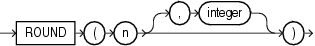ROUND (number)

Syntax

round_number::=Description of the illustration round_number.eps

Purpose

ROUND returns n rounded to integer places to the right of the decimal point. If you omit integer, then n is rounded to zero places. If integer is negative, then n is rounded off to the left of the decimal point.

n can be any numeric data type or any nonnumeric data type that can be implicitly converted to a numeric data type. If you omit integer, then the function returns the value ROUND(n, 0) in the same data type as the numeric data type of n. If you include integer, then the function returns NUMBER.

ROUND is implemented using the following rules:

1. If n is 0, then ROUND always returns 0 regardless of integer.

2. If n is negative, then ROUND(n, integer) returns -ROUND(-n, integer).

3. If n is positive, then

ROUND(n, integer) = FLOOR(n * POWER(10, integer) + 0.5) * POWER(10, -integer)

ROUND applied to a NUMBER value may give a slightly different result from ROUND applied to the same value expressed in floating-point. The different results arise from differences in internal representations of NUMBER and floating point values. The difference will be 1 in the rounded digit if a difference occurs.

• for more information on how Oracle Database handles BINARY_FLOAT and BINARY_DOUBLE values

• FLOOR and CEIL, TRUNC (number) and MOD for information on functions that perform related operations

Examples

The following example rounds a number to one decimal point:

SELECT ROUND(15.193,1) "Round" FROM DUAL;

Round
----------
15.2

The following example rounds a number one digit to the left of the decimal point:

SELECT ROUND(15.193,-1) "Round" FROM DUAL;

Round
----------
20